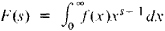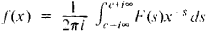# Mellin Inversion Formula

The following article is from The Great Soviet Encyclopedia (1979). It might be outdated or ideologically biased.

## Mellin Inversion Formula

an invertible transformation of functions given by the formulasandIt is used in some problems in analysis and in analytic number theory. First noted by the German mathematician B. Riemann in 1859, it was examined in detail by the Finnish mathematician R. H. Mellin in 1902.